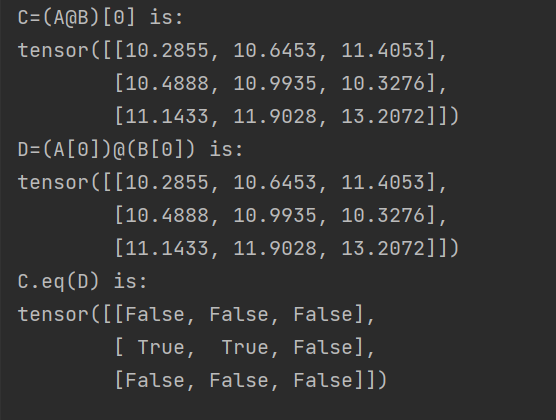# Two tensors produced from the '@' operation are not eqaul under the measurement of '.eq()'

Hi, I’m quite new to PyTorch and I came cross the ‘@’ operation, which does matrices multiplication for the last two dimensions. (referrence: How does the @ sign work in this instance?).

As far as I understand, given two tensors A (bsz, d1, d2), and B (bsz, d2, d3), (A@B)[i] should equal to (A[i])@(B[i]); thus, these two results from ‘@’ should be identical when call the ‘.eq()’ measurement (a simple code exmaple is shown below with i=0).

``````import torch
torch.set_printoptions(profile="full")

A = torch.rand(16, 3, 40)
B = torch.rand(16, 40, 3)

C = (A@B)
D = (A)@(B)

print('C=(A@B) is:')
print(C)
print('D=(A)@(B) is:')
print(D)
print('C.eq(D) is:')
print(C.eq(D))
``````

However, what I got from the ‘C.eq(D)’ line was not as I expected to be all ‘True’, and the printed values of C, D are identical as I expected.I understand comparing the values with our raw eyes is not the best way to decide whether two tensors are equal, but the results of ‘.eq()’ is really confusing.

And I find that when I declare A, B to be integers, C=(A@B) always equals to D=(A)@(B) under ‘.eq()’ measurement, so here are my questions:

1. why C.eq(D) is not all ‘True’ when declaring A, B to be float? And is all ‘True’ if A, B declared as integers?

2. Regarding Q1, I suspect it has something to do with the datatypes, however I can’t find anything related to this difference on PyTorch Docs, help on this perspective is very much appreciated!

3. Regaiding Q3, if the ‘.eq()’ behaviour is caused by the nature of datatype float vs. integer, why the printed values, shown in the picture, are the same from raw eye observation (especially after I called: torch.set_printoptions(profile=“full”))?

I’m using PyTorch 1.12.0 and thanks in avdance!

Hi Alex!

Because of floating-point round-off error, this will not be true in general.

The two computations are mathematically equivalent, but pytorch chooses
to perform various arithmetic operations in differing orders that can lead to
differing round-off error.

Integer operations don’t suffer the same round-off error that floating-point
operations do.

You will need to print the values out to close to machine accuracy to see the
difference. Try `torch.set_printoptions (precision = 20)`.

Best.

K. Frank

Hi Frank!

Thank you very much on the explanation of the round-off error, and I indeed noticed the difference after torch.set_printoptions (precision = 20).

However I’m still a little confused about the statement that: “pytorch chooses to perform various arithmetic operations in differing orders that can lead to differing round-off error”. I agree that the different in the orders of operations can lead to different round-offs; thus, inequality when calling ‘.eq()’, but I can’t understand why processing in batch can lead to such differences (the code below is a pseudocode that I think how ‘@’ deals with batch).

``````given: A=(bsz, d1, d2), B=(bsz, d2, d3), we are to calculate A@B
init: C=()
for i in (1, 2, ..., bsz):
C.append(A[i]@B[i])
return C
``````

From my understanding, I think when ‘@’ deals with batching, it just computes ‘A[i]@B[i]’ bsz times and stacks the results together, and thus should yield identical outcomes for ‘A[i]@B[i]’ and ‘A@B[i]’. However as this is not the truth in practice, can you please tell me where I got it wrong on how ‘@’ deals with batching? Thx in advance!

Hi Alex!

Conceptually this is a good way to picture what is happening, but
it doesn’t match the exact details of pytorch’s actual computation.

Under the hood pytorch has a lot of optimized code to make sure
it uses the floating-point pipelines as efficiently as possible. (Note,
modern cpus have floating-point pipelines, so these comments apply
to cpus as well as gpus.)

The pytorch code will often reorder the computation in order to keep
the floating-point pipelines as full as possible when, for example, the
size of the input changes. In your example, you are moving from a
batch matrix multiply to a single matrix multiply. This could easily
trigger such a reordering.

You will also see this kind of reordering when you move from the cpu
to gpu, when you move from one hardware platform to another, when
you change pytorch versions, and so on. (You will also see this when
you move from single precision to double precision, although this is less
likely to be noticed because the single-precision and double-precision
results won’t be exactly the same even when the computations are
performed in the same order.)

Best.

K. Frank

Hi Frank,

Thank you very much for your responsive and detailed reply! Now I get to know a little deeper about the mechanics of how the computation is done, which is very helpful for me to understand some other inconsistencies!